Cantor never bores (1)

Given a set of countable sets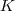, such thatis totally ordered by inclusion, videlicet for every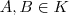either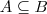or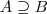. Intuitively, for at every step in this chain one element at least must be added, one expects the setto be countable as well.

Supposeis countable. Then the union,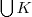is a countable union of countable sets, hence countable. (Suppose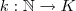is an enumeration ofandenumerations of the elements of the chain. Thenenumerates.)

Thusis an upper bound of. In the poset of countable subsets of some set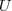, of whichis a subset, every non-empty chain has an upper bound. Hence, using Zorn’s lemma there is a maximal element, say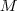.

Supposeis uncountable, then there exists a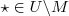.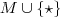is most definitely also countable and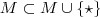which contradicts’s maximality. We are forced to conclude that there exists an uncountable chain of countable sets.

Cantor’s set theory keeps surprising.

Update: an example of such a chain is the set of the countable ordinals.

Another update: a “more concrete” example are the downsets in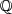without the empty set anditself. These downsets correspond to real numbers, see Dedekind Cuts.